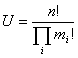Download UniquePermutation.zip The numbers of permutations on a set of n elements is n! (see definition). or example permutations of the word “ask” are:

ask, aks, sak, ska, kas, ksa

We often need to obtain unique permutations over a given set programmatically. Take the word “good” for example, the unique permutations are:

good, godo, gdoo, ogod, ogdo, oogd, oodg, odgo, odog, dgoo, dogo, doog

And we can prove mathematically that the total number of the permutations is :where n is the length of the sequence and m is the number of a non-unique letter. In the example given above, n = 4 and i = 1 (only one letter “o” is non-unique and it has two o’s, so m = 2 and 4!/2! = 12. The following is the C# code I wrote to achieve this:

class Program {

public ArrayList Unique(string s) {

char[] c = s.ToCharArray();

ArrayList ayList = new ArrayList();

for (int i = 0; i < c.Length; i++)

return ayList;

}

public ArrayList Permute(string s) {

ArrayList tmpList = new ArrayList();

ArrayList permList = new ArrayList();

if (s.Length == 1) {

return permList;

} else {

ArrayList list = Unique(s);

foreach (char c in list) {

for (int i = 0; i < s.Length; i++) {

string tempS = string.Empty;

if (s.Substring(i, 1) == c.ToString())

tempS = s.Remove(i, 1);

else

continue;

tmpList = Permute(tempS);

foreach (string t in tmpList) {

if (!permList.Contains(c.ToString() + t))

}

}

}

}

return permList;

}

static void Main(string[] args) {

Program p = new Program();

ArrayList ayList = p.Permute("good");

foreach (string s in ayList) Console.WriteLine(s);

Console.WriteLine();